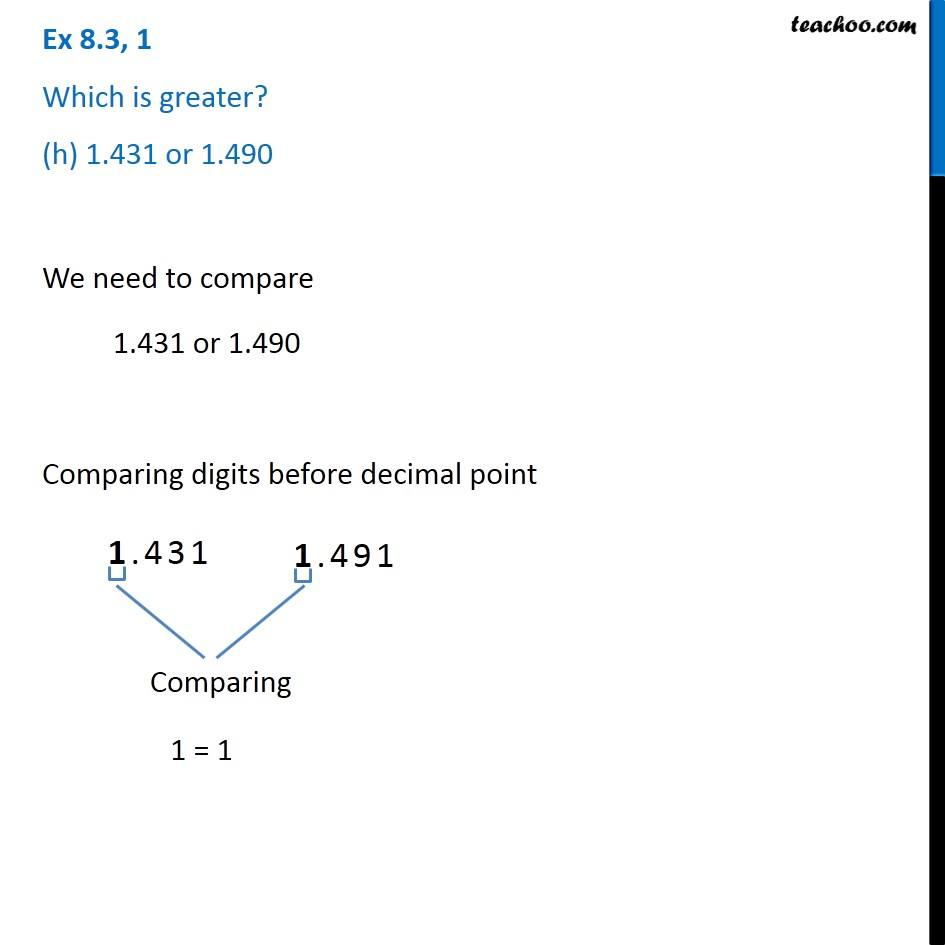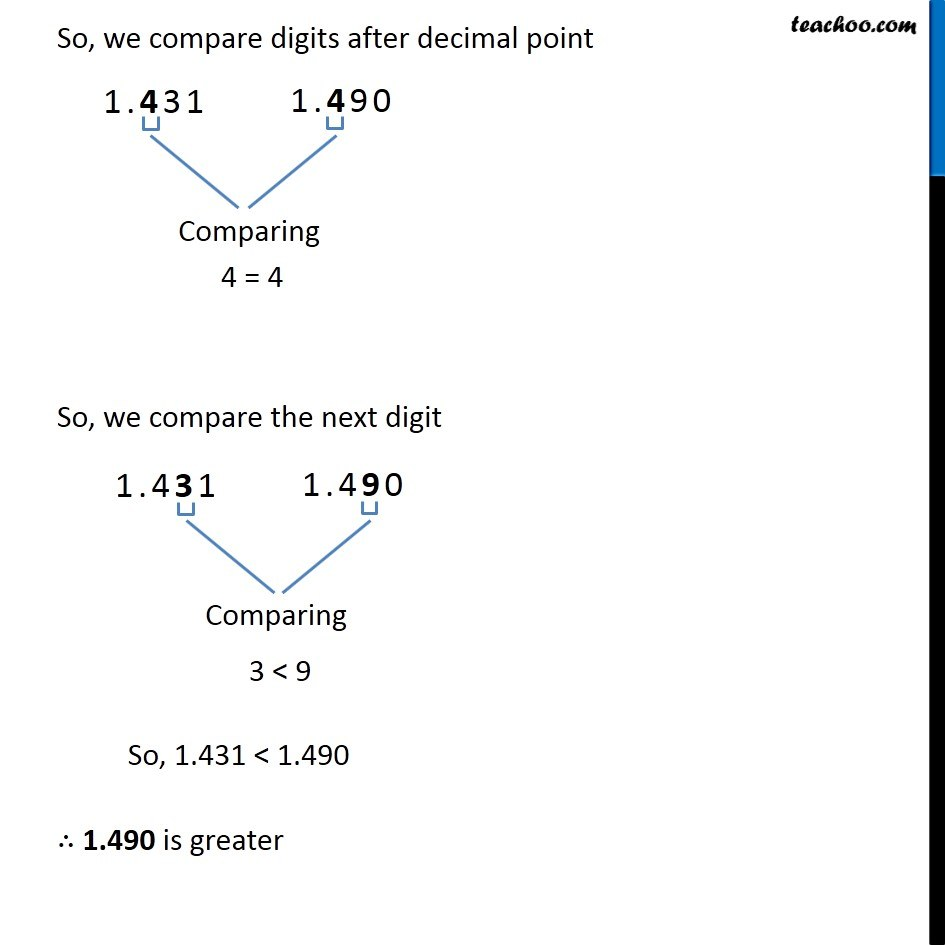1. Chapter 8 Class 6 Decimals
2. Concept wise
3. Comparing decimals

Transcript

Ex 8.3, 1 Which is greater? (h) 1.431 or 1.490 We need to compare 1.431 or 1.490 Comparing digits before decimal point 1 = 1 So, we compare digits after decimal point 4 = 4 So, we compare the next digit 3 < 9 So, 1.431 < 1.490 ∴ 1.490 is greater

Comparing decimals